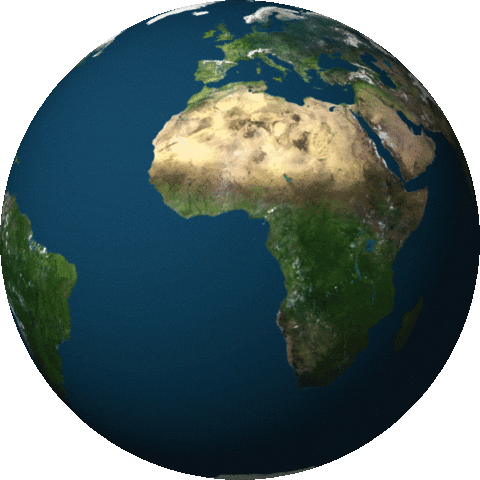# What is the circumference of the earth in km?

Dec 31, 2015

$40 , 074.16$ $k m$

#### Explanation:

The earth has a diameter of $12 , 756$ $k m$.

Circumferenceis $\pi d$

$= \pi \left(12 , 756\right)$

$= 40074.1558892$

$= 40 , 074.16$ $k m$http://giphy.com/gifs/earth-FrOlhISiIhAFa